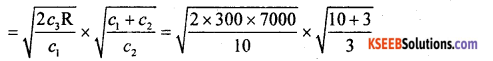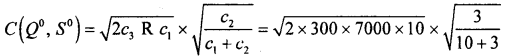# 2nd PUC Statistics Previous Year Question Paper March 2016

Students can Download 2nd PUC Statistics Previous Year Question Paper March 2016, Karnataka 2nd PUC Statistics Model Question Papers with Answers helps you to revise the complete Karnataka State Board Syllabus and score more marks in your examinations.

## Karnataka 2nd PUC Statistics Previous Year Question Paper March 2016

Time: 3 Hrs 15 Min
Max. Marks: 100

Section-A

I. Answer any TEN of the following questions: (10 × 1 = 10)

Question 1.
What is a life table?
Life table is a tabular presentation of numerical data describing the mortality experience of a cohort.

Question 2.
Define an ‘Index number’.
Index number is a statistical measure, designed to show an average change in a variable or group of related variables with respect to time, geographical area or income group etc.

Question 3.
Write the formula for computing Marshall-Edgeworth’s quantity Index number.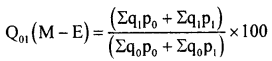Question 4.
What is a histogram?
The graph of actual values of time series is called Histogram.

Question 5.
What are the values that a Bernoulli variate can take?
0,1.Question 6.
Name the distribution for which standard deviation and variance are equal.
Standard Normal distribution.

Question 7.
What is confidence coefficient?
The probabilty that the confidence interval contains the parameter is called confidence coefficient (1 – α).

Question 8.
Define critical region.
The set of all those values of the test statistic, which leads to the rejection of the bull hypothesis, is called critical region (w).

Question 9.
Write the degrees of freedom while testing independence of attributes in a 2 x 2 contingency table.
1 degrees of freedom.

Question 10.
What is chance cause of variation?
A small amount of variation for which no specific cause can be observed in the quality of the product, this variation is called as ‘chance causes’.

Question 11.
Define ‘Solution’ in an LPP.
A set of real value X = (x1,x2,……….xn), which satisfies the constraints A X(≤ = ≥)b, is called a solution.

Question 12.
What is a two-person zero sum game?
In a game of two players, the gain of one player is the loss of the other is called two – person zero – sum game.

Section – B

II. Answer any TEN of the following questions : (10 × 2 = 20)

Question 13.
There was a reord of 4000 live births in a city in a year. The number of neonatal deaths was 100. Calculate the neonatal mortality rate.
Neo-natal mortality rate. (N. M. R)N.M.R = $$\frac { 100 }{ 4000 }$$ × 1000 = 25.

Question 14.
Write any two limitations of index numbers.

• Many formulae are ased and they give different answers for the index.
• As the customs and habits change from time to time, the uses of commodities also vary.

Question 15.
If PL01 = 105 and PP01 = 125, then find PDB01.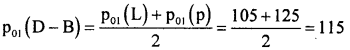Question 16.
Mention all the components of a time series.

• Secular trend
• Seasonal variation
• Cyclical variation
• Irregular/Random variation.

Question 17.
Define interpolation and extrapolation.
‘Interpolation’ is the technique of estimating the value of the dependent variable (Y) for any intermediate value of the independent variables (X). Extrapolation is the technique of estimating the value of dependent variable (Y) for any value of the independent variable (X) which is outside the range of the given series.Question 18.
For a chi-square variate with 9 degrees of freedom, obtain mean and mode of the distribution.
Given : n = 9; Mean = n = 9; Mode = (n – 2) = 9 – 2 = 7.

Question 19.
Write the values of β1, and β2 in a t-distribution.
For a t-distribution β1, = 0 (Non – skewed) and β2 > 3 (Lepto Kurtic).

Question 20.
Compute SE(p1 – p2), given n1 = 80, n2 = 40, P1 = 0.8 and P2 = 0.4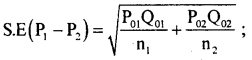Here P1= P01 ; P2 = P02 and Q01 = 1 – P01 = 1 – 0.8 = 0.2, Q02 = 1 – P02 = 1 – 0.4 = 0.6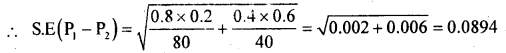Question 21.
Define Null and Alternative hypothesis.
Null hypothesis is a hypothesis, which is being tested for possible rejection, assuming that it is true. (H0) null hypothesis is rejected, is called alternative hypothesis (H1).

Question 22.
Compute the lower and upper control limits for X̄-chart when X̄1 =40, σ1 = 6 and A = 1.342
L.C.L = X̄1 – Aσ1 = 40 – (1.342 × 6) = 31.948 U.C.L = X̄1 + Aσ1 = 40 + (1.342 × 6) = 48.052

Question 23.
The objective function and two solutions of an LPP are Max Z = 200x: + lOOy and A(0,18); B (12,0). Find the optimal solution.
ZA = 200(0)+100(18)= 1800, ZB = 200 (12)+100(4) = 400
ZB is maximum and so, the optimal solution is at B: n = 12, y = 0.

Question 24.
Define pure and mixed strategy in a game.
Pure strategy of a player is the pre-determined decision / Rule to adopt a specified course of action irrespective of the course of action of the opponent.

Mixed strategy of a player is his pre-decision to choose his course of action according to certain pre-assigned probabilities.Section – C

III. Answer any EIGHT of the following questions : (8 x 5 = 40)

Question 25.
Compute the net reproduction rate from the following data :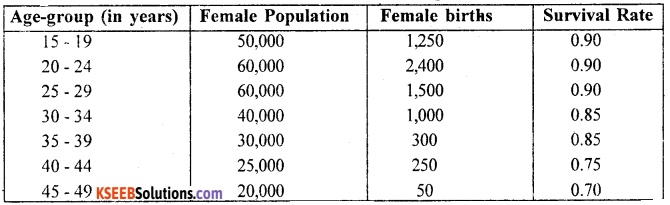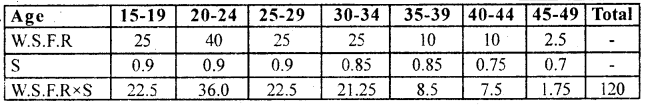W.S.F.R – Women specific fertility rate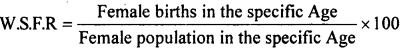W.S.F.R(15 -19) = $$\frac { 1250 }{ 50,000 }$$ × 100 = 25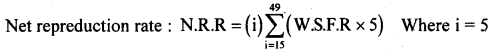N.R.R = 5 × 120 = 600.

Question 26.
Calculate weighted GM price index number for the year 2014 from the following data.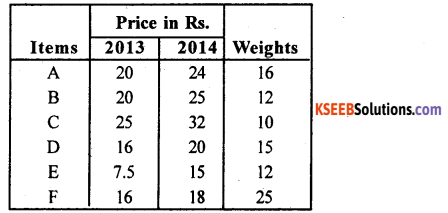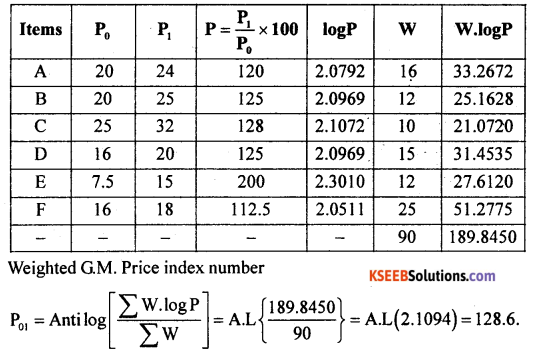Question 27.
What is a consumer price index number? Write any four of its uses.
Cost of living index number/consumer price index number is the index number of the cost met by a specified class of consumers in buying a basket of goods and services.
Uses:

• It is commonly used in fixation of salary, dearness allowances to the employees.
• They are used by govt, in formulation of price policy, wage policy and general economic policies.
• They are used in evaluation of purchasing power of money and deflating the money.
• They are used for comparing cost of living of different classes of people.

Question 28.
Compute 4 – year moving averages from the following data :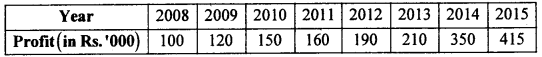Let X and Y be the year and profit.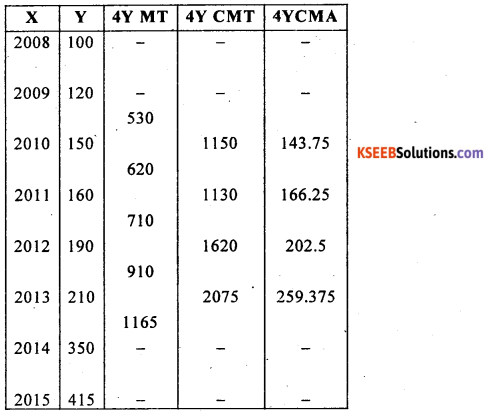Here
4YMT-4 yearly moving total.
4YCMT – 4 yearly centered moving total.
4YCMA – 4 yearly centered moving average.Question 29.
Interpolate the number of workers earning wages Rs. 775 per day from the following table.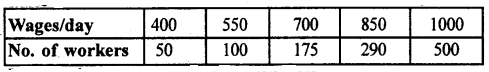Let X and Y Wages and no.of workers.
Here the no. of Known values of Y are n = 15 , so prepare leading differences upto Δ4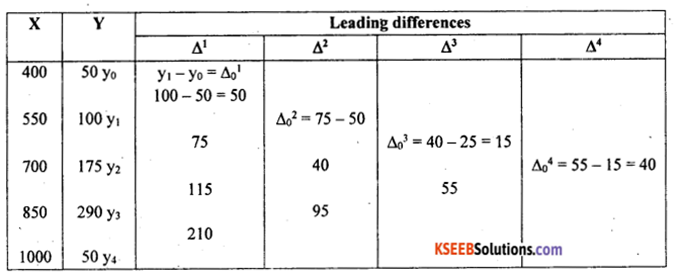Here ‘Y’ value to be determine for X = 775. The Newton’s formula of interpdation is.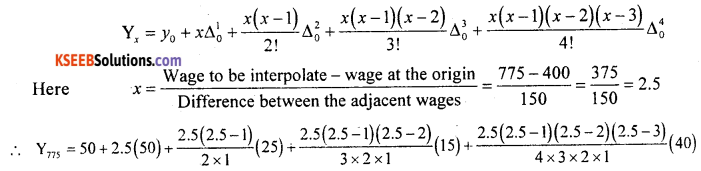= 50 + 125 + 46.875 + 4.6875 – 1.5625
Y775 = 225 are the number of workers earns wages ₹.775 per day.

Question 30.
40% of the buttons manufactured by a firm are found to be defective. In a random sample of six buttons, find the probability of getting.
(i) exactly 4 defective buttons
(ii) more than one defective button.
Let X be the number of buttons manufactured is a Binomial.
Variate with parameters n = 6 and p = 40% = 0.4, so q = 1 – p = 0.6. Then the probability mass function is:
p (x) = nCx px qn-x= 0, 1, 2 ……. n.
= 6Cx (0.4)x (0.6)6-x; x = 0, 1, 2 6.
(i) P(exactly 4 defective buttons) = p(x = 4)
= 6C4 (0.4)4 (0.6)6-4 = 15 × 0.0256 × 0.36 = 0.1382

(ii) P(more than 1 defective button) =p(x > 1) = 1 -p(x = 0)
= 6C0 (0.4)0 (0.6)6-0 = 1.1. 0.466 = 0.0466.

Question 31.
There are 20 marbles in a box, out of which 12 are red coloured and the rest white coloured. A child picks 6 marbles at random. Find the probability that the child gets 4 red coloured marbles.
Let x be the number of red coloured marbles picked is a Hypergeometric variate with the parameters a = 12 (Red), b = 20 – 12 = 8 (White) and n – 6 (picked, then the p.m.f is:-)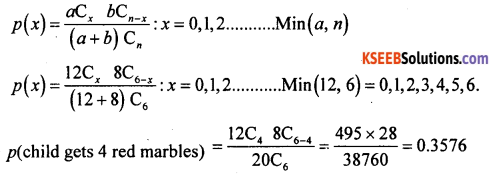Question 32.
Among 400 randomly -selected persons of a city, 280 like ice-cream. Test at 1% level of significance that 50% of the people in that city like ice-creams.
Linking ice-cream is an attribute, so use test for proportion.
Given: n = 400, x = 280 (ice cream liked)
∴ Sample proportion : p $$\frac{x}{n}=\frac{280}{400}$$ and
P0 = 0.5 (50% people like ice cream): α = 1%
H0: 50% people like ice cream (i.e., P0 = 0.5)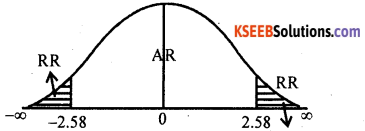H1 : people liking ice cream differ from 50% (i.e., P0 ≠ 0.5 ) {two tailed test ±K)
under H0 the test statistic is :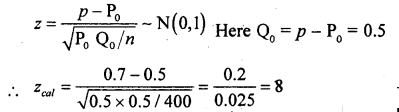At α = 1% the two tail crtical values are ±K = ± 2.58
Here Zcal lies in Rejection Region (R.R)
∴ H0 is rejected and H1 is aceepted.
conclusion: P0 ≠ 0.5 : people liking ice cream differ from 50%.

Question 33.
From the following, test whether mean of the first population is greater than the mean of the second population. (Use α = 5%)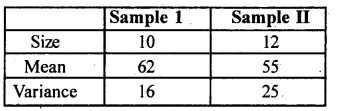Given: n1 = 10, n2 = 12, x̄1 = 62, x̄2 = 55, S12 = 16 and S22 = 25 (S2 sample variance). Here n1, n2 < 30 use small sample, t-test for equality of means, α = 5%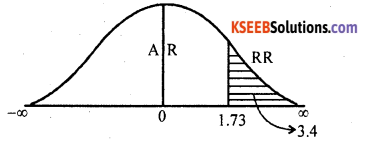H0: Means of first and second population are same (i.e., µ1, = µ2)
H1 : Mean of first population is greater than mean of second population (i.e., µ1 > µ2) {upper tail test ±k}
under H0 the t-test statistics is:-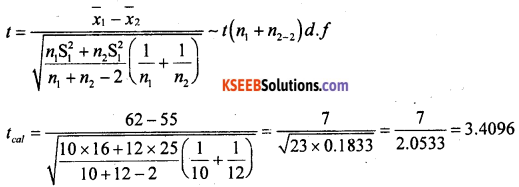At α = 5% for (n1 + 2-2) = 10 + 12 – 2 = 20 d.f the upper tail critical value k = 1.73
Here tcal lies in rejection region (R.R)
∴ H0 is rejected and H1 is accepted.
Conclusion: Mean of first population is greater than second population (µ1 > µ2)Question 34.
Twenty rolls of different types of cloth contained the following number of defects in each roll : 2, 4, 2, 3, 5, 6, 7, 2, 3, 6, 4, 8, 2, 4, 5, 2, 3, 1, 2, 4.
Calculate the control limits for C-chart.
7; SC
Standard is not known, for c-chort we need $$\overline{\mathrm{C}}=\frac{\Sigma \mathrm{C}}{k}$$ = C – no. of defects and k = 20 rolls.
∴ C̅ = $$\frac { 75 }{ 20 }$$ = 3.75. The control limits are:-
C.L = C = 3.75;
L.C.L = $$\overline{\mathrm{C}}-3 \sqrt{\overline{\mathrm{C}}}=3.75-3 \sqrt{3.75}$$ = 3.75 – 5.8094 = -2.0594 = 0
U.C.L = $$\overline{\mathrm{C}}+3 \sqrt{\overline{\mathrm{C}}}=3.75+3 \sqrt{3.75}$$ = 9.5594.

Question 35.
For the following transportation problem, find the initial basic feasible solution by Matrix Minima Method and obtain the cost associated with the solution.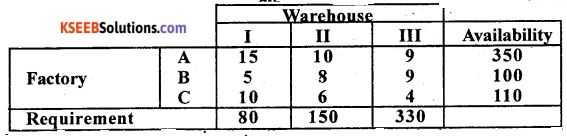By matrix minimum method, the first allocation is made at the least cost cell as below: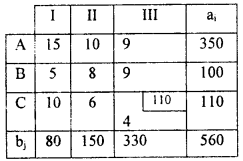Here Σa 1 = 560 = Σbj T.R is balanced.
Lest cost is 4 at (3, 3), allocated as: X33 = min (110,330) = 110, ‘C’ is satisfied So, delete and replace 330 by (330 -110) = 220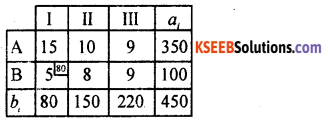Next least cost is 5 at (2, 1) allocate as: X21 = min (100, 80) = 80. 1 is satisfied and delete. Replace 100 by (100 – 80) = 20.
Next reduced table is: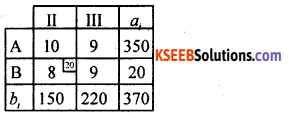The least cost in the reduced cost matrix is 8. Allocate as:- X22 = min (20, 150) = 20. B is satisfied and delete, replace 150 by (150 – 20) = 130.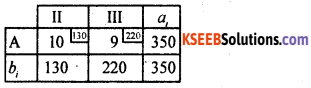Next allocate at (1,3) as X13 = min (350,220) = 220. Ill satdisfied and the last allocation of the remaingcell is: X12 = min (350-220,130) = min (130,130)= 130.
The suggested initial basic feasible solution and its cost is:-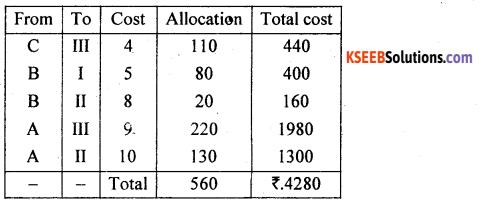Question 36.
A machine costs Rs. 8,000. Its maintenance cost and resale value per year are given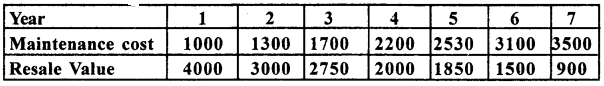Determine the optimal replacement age of the machine.
Given: P = 8000, the given data can be written as bellow: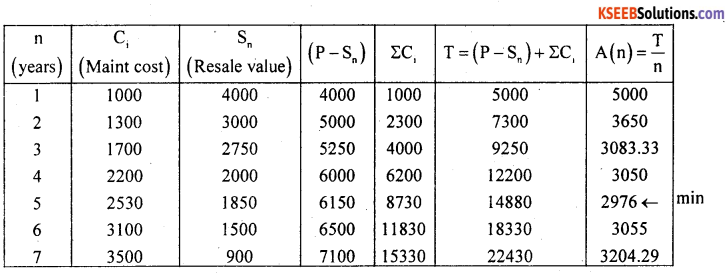From the above table the A(n) – Annual average maintenance cost is minimum for the year n = 5 is the optimal replacement age of the machine. A(5) = ₹ =2976.

Section – D

IV. Answer any TWO of the following questions : (2 × 10 = 20)

Question 37.
From the following data calculate the Standardised Death rate. Hence find the locality with healthier population.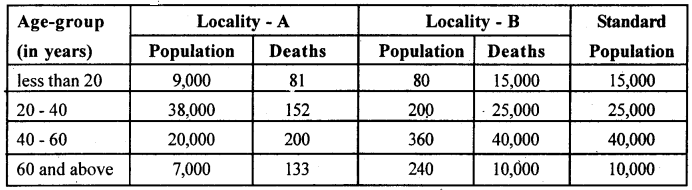Let A, B – be the Age specific death rates of locality A and locality B and Ps be the standarc population.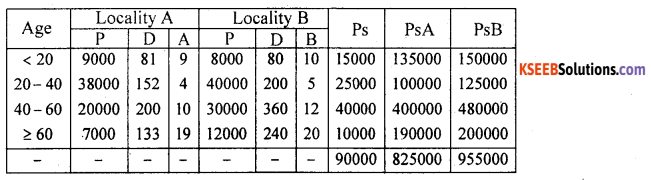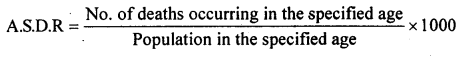For locality A: As (OR (< 20) = $$\frac { 81 }{ 9000 }$$ × 1000 = 90, similarly can be calculated for other age groups.
For locality B: A.S.D.R (< 20) = $$\frac { 80 }{ 8000 }$$ × 1000 = 10, similarly can be calculated for other age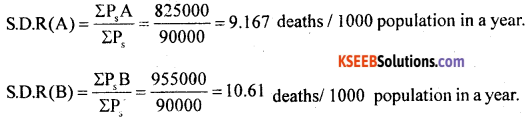groups.
Conclusion: Here S.D.R. (A) < S.D.R (B), so locality A is healthier population than localite B.Question 38.
For the following data, compute Fisher’s price Index number. Show that Fisher’s Index number satisfies Time reversal and Factor reversal tests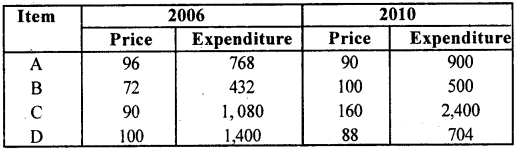Expenditure – Base year = p0 q0, current year – p1 q1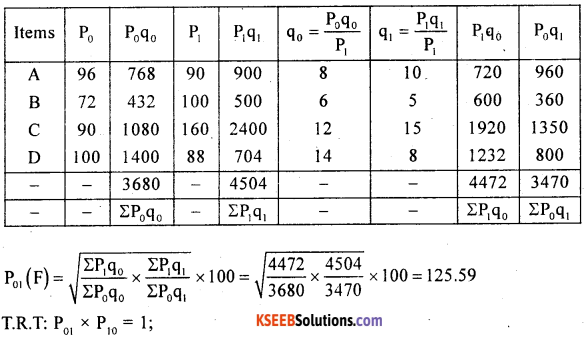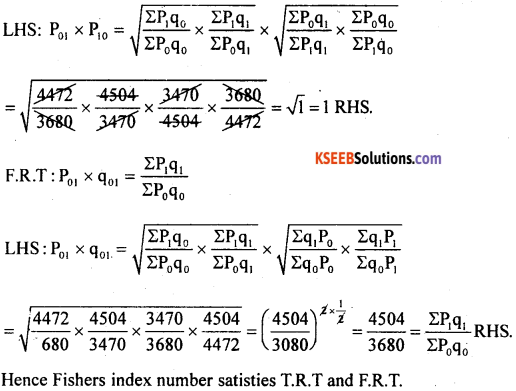Question 39.
Find a parabolic trend of the form Y = ax + bx + cx2 for the following time series.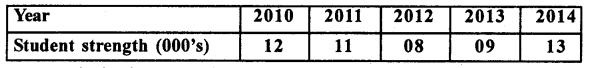Let x and y be the year and students strength.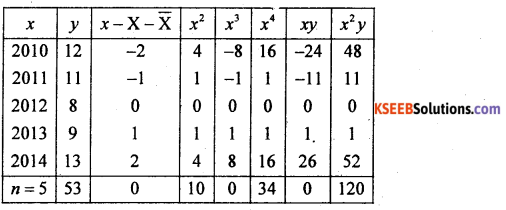Here X̄ = middle most year = 2012
From the normal equation: na + b Σx + c Σx2 = Ey
5a + b.0 + c.10 = 53; 5a + 10c = 53 ………. (1)
From: a Σx + b Σx2 + c Σx3 – Σxy; a.0 + b.10 + c.0 = 0
10 b = 0 ∴ b = 0.
And from: a Σx2 + b Σx3 + c Σx4 = Σx2y; a. 10 + b.0 + c.34 = 120
i. e., 10a + 34c = 120 …….(2)
From (1) and (2)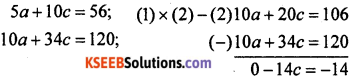∴ c = 1. put c = 1 in (1) we get 5a +10 (1) = 53, 5a = 53 – 10
a = $$\frac { 43 }{ 5 }$$ = 8.6.
The fitted parabolic trend equation is: ŷ = a + bx + cx2
ŷ = 8.6 + 0x + 1x2; ŷ = 8.6 + x2Question 40.
Fit a Poisson distribution to the following data and test the goodness of fit at 5% level of significance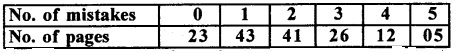Let X be the number of mistakes is a poisson variate with λ is obtained as below:- let f be the no of pages, then from the frequency disribution: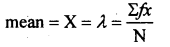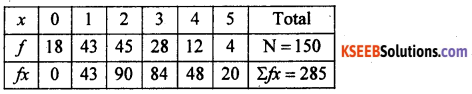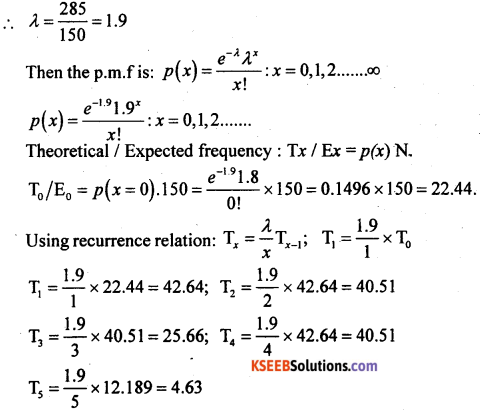The fitted theoretical and observed frequency distribution is:- (Approximated)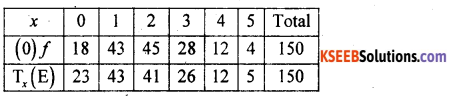χ2 -TEST: H0: poisson distribution is good fit (0i = Ei)
H1: poisson distribution is not a good tit (0i ≠ Ei
Under H0, the χ2-test statistic is:-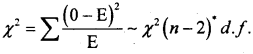Here λ is estimated from the data and so d.f. will be (n – 1 – 1) = (n – 2)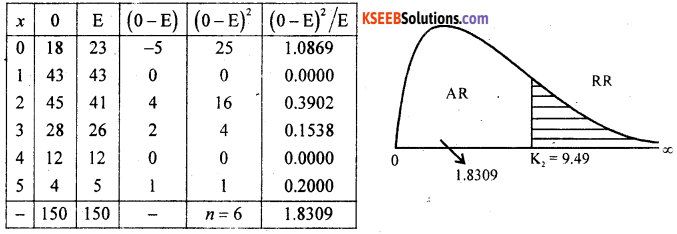∴ χcal2 = 1-8309
At α = 5% for (n – 2) = 6 – 2 = 4d.f the upper tail critical value k2 = 9.49.
Here χcal2 lies in acceptance region.
∴ H0 is accepted
Conclusion: Poisson distribution is good fit (0i = Ei).

Section – E

V. Answer any TWO of the following questions: (2 × 5 = 10)

Question 41.
The distribution of monthly income of Rs. 5,000 workers of a factory follows Normal distribution with mean Rs. 10,000 and standard deviation Rs. 1,000. Find the probability of the number of workers.
(i) having income more than Rs. 9,000
(ii) having income in between Rs. 8,500 and Rs. 12,000.
Let x be the monthly income is a normal variate with the parameters μ= 10,000 and σ = 1,000 and N = 5000. Then S N.V is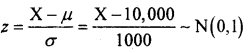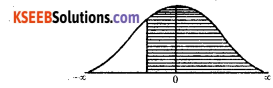(i) P(income more than ₹9000) = P(X > 9000)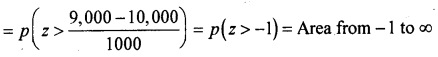No. of workers = 0.8413 × 5000 = 4206.5

(ii) P(income between ₹ 8500 and ₹ 12,000) = p(8500 < x < 12000)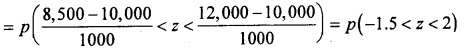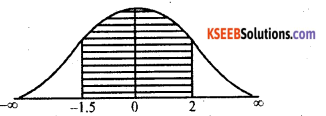= Area from (-1.5) to ∞ – Area from 2 to ∞
= 0.9332 – 0.0228 = 0.9104
No. of Workers = 0.9104 × 5000 = 455.2.

Question 42.
Samples of electric lamps manufactured by two firms gave the following results :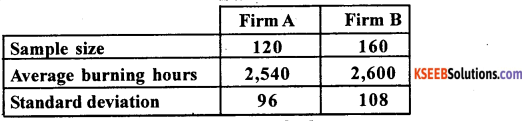Test at 5% level of significance whether the average life of bulbs manufactured by firm A is les than the average life of bulbs manufactured by firm B.
Given: n1, = 120, n2 = 160, x̄1 = 2540, x̄2 = 2600 S1 = 96, S2 = 108, α = 5%
H0: average life of firm A and firm B is same (i.e., μ1 = μ2)
H1 : Average life of firm A is less than firm B (i.e., μ1 < μ2) {Lower tail test}
Under H0, the test statistic is: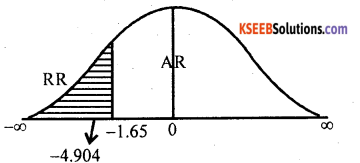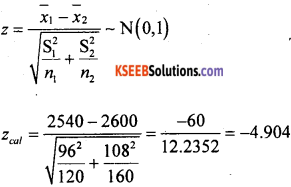At α = 5% the lower tail critical value -k = – 1.65
Here Zcal lies in rejection region (R.R)
∴ H0 is rejected and H1 is accepted.
conclusion: Average life of lamps of firm A is less than firm B lamps (i.e., μ1 < μ2).Question 43.
A normal variate has variance 100. Twenty random observations of the variate has variance 144. Test at 1% level .of significance whether the variance of the population is 100.
Given: σ2 = 100: n = 20, S2 = 144, a = 1%
H0: population variance is 100 (i.e., σ2 = 100)
H1 : population variance differs from 100 {i.e., σ2 ≠ lOO) (two tailed test)
Under H0, the χ2 – test statistic is:-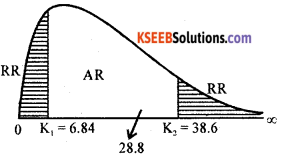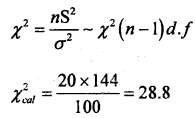At α = 1% for (n – 1) = 20 – 1 = 19 d.f the two tail critical values are k1 = 6.84 and k2 = 38.6
Here χcal2 lies in acceptance region (A.R)
∴ H0 is accepted.
Conclusion: Population variance = 100 (i.e., σ2 = 100).

Question 44.
The demand for an item is 7,000 units per year. The cost of placing an order is Rs. 300 per year. The holding cost is Rs. 10 per unit per year. The shortage cost is Rs. 3 per unit per year. Find
(i) Economic Order Quantity
(ii) Minimum annual average inventory cost.